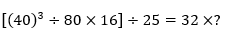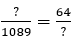Dear Aspirants,

Quantitative Aptitude Quiz For SBI PO/Clerk Prelims

Numerical Ability or Quantitative Aptitude Section has given heebie-jeebies to the aspirants when they appear for a banking examination. As the level of every other section is only getting complex and convoluted, there is no doubt that this section, too, makes your blood run cold. The questions asked in this section are calculative and very time-consuming. But once dealt with proper strategy, speed, and accuracy, this section can get you the maximum marks in the examination. Following is the Quantitative Aptitude quiz to help you practice with the best of latest pattern questions.

Directions (1-5): Use the data given in the table and answer the questions.
Note: The ratio of failed boys to girls is reverse of that of passed.Q1. If in school A, 100 girls are failed in the test. Find the total strength of A.

1100
1300
1200
1400
1000

Q2. Find the ratio of no. of boys passed from A to that of B.
4 : 5
5 : 4
6 : 5
5 : 6
3 : 5

Q3. Passed girls from D is what percent of passed girls from B.
625/2%
625/9%
625/3%
627/4%
625/6%

Q4. If the difference between the failed boys to failed girls is 25 in school D. Find the ratio of passed to failed students from D.
3 : 1
4 : 1
2 : 1
1 : 2
3 : 2

Q5. Find the average number of passed students from schools A, B and C together.
1200
3000
1250
1000
1800

Directions (6-10): Study the following table and answer the following questions.Q6. Total imports in year 2006 is approximately what percent of total imports in all the years together?

21%
29%
17%
18%
25%

Q7. The rise in electronic items import in 2007 from 2006 was nearly (in percentage)?
15%
18%
25%
22%
11%

Q8. Over the 4 year period from 2006 to 2009 the electronic import income is approximately what percent of total import income during same period?
20%
11%
15%
29%
25%

Q9. If the electronic items have not been imported in the year 2006 them what will be the total imports of that year?
Rs 5470 Cr.
Rs 5830 Cr.
Rs 5970 Cr.
Rs 5770 Cr.
Rs 6404 Cr.
Solution:
In 2006 total imports = Rs 6404 Cr.
If electronic items have not been imported, then the total imports of that
Year = 6404 – 634 = Rs 5770 Cr.

Q10. What is the average import of total items from China from the year 2005 to 2008?
Rs 6121.25 Cr
Rs 6221.25 Cr.
Rs 6083.25 Cr.
Rs 6112.25 Cr.
Rs 6234.25 Cr

Directions (11-15): What will come in place of (?) in the following questions?

Q11. 621 × ? × 6 = 152766

39
43
47
41
46

Q12.12
15
18
21
16

Q13. 6784 + 2213 + 844 - ? = 6743 + 775
2332
2323
2343
2442
2424

Q14. 11960 ÷ ? = 65 × 23
6
11
3
8
12

Q15.264
258
236
244
246

You May also like to Read: